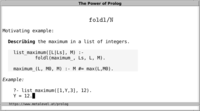# Higher-order Predicates

Prolog predicates that take predicates as arguments are called higher-order predicates, or meta-predicates.

 Video:## call/N

The call/N family of built-in predicates allow us to call Prolog partial goals dynamically. A partial goal is a term that denotes a Prolog goal to which zero or more arguments are added before it is called.

The mechanism to invoke arbitrary Prolog goals dynamically is called meta-call, and it is the basic building block by which we can define arbitrary meta-predicates in Prolog.

Importantly, the call/N family of predicates retain logical purity of the predicates they call.

Like most meta-predicates, call/N could in principle be implemented in Prolog itself. For example, we could implement call/1 as follows, using one clause for each available predicate:
```call(true).
call(X #= Y)  :- X #= Y.
call(call(G)) :- call(G).
etc.
```
Of course, this would need an impractical number of clauses in practice, and for this reason the call/N family of predicates is implemented differently.

## maplist/N

The predicates maplist/2 and maplist/3 are among the most important and most frequently used meta-predicates. All widely used Prolog systems provide them as built-in or library predicates.

The goal maplist(Pred_1, Ls) is true iff call(Pred_1, L) is true for each element L in the list Ls.

The goal maplist(Pred_2, As, Bs) is true iff call(Pred_2, A, B) is true for each pair of elements A∈As and B∈Bs that have the same index.

There are further predicates in this family (maplist/[4,5,...]) that are less frequently used.

If your Prolog system does not provide these predicates, you can easily define them yourself. For example, maplist/3 is declaratively equivalent to:
```maplist(_, [], []).
maplist(Pred_2, [A|As], [B|Bs]) :-
call(Pred_2, A, B),
maplist(Pred_2, As, Bs).
```
Using goal expansion, the meta-call can be compiled away in many cases to improve performance.

The primary advantage of maplist/N is that you can lift any relation that holds for a single element (or single pair, triple, etc. of elements) to lists of such elements (or corresponding elements).

For example, we can state that a term E is different from all elements in Ls:
```?- maplist(dif(E), Ls).
Ls = []
;  Ls = [_A], dif:dif(E,_A)
;  Ls = [_A,_B], dif:dif(E,_A), dif:dif(E,_B)
;  Ls = [_A,_B,_C], dif:dif(E,_A), dif:dif(E,_B), dif:dif(E,_C)
;  ... .
```
This works in all directions, even if Ls is not instantiated.

## if_/3 and related predicates

The meta-predicate if_/3 is an important recent development that is described in Indexing dif/2 by Ulrich Neumerkel and Stefan Kral.

Implementations are available for:
• SICStus Prolog: library(reif)
• Scryer Prolog ships with library(reif). Post ?- use_module(library(reif)). to use if_/3 and related meta-predicates.
• SWI-Prolog: library(reif)
Importantly, if_/3 combines desirable declarative properties with good performance in many situations of high practical relevance.

For example, the predicate hailstone/2 that is described in Basic Concepts can be expressed with if_/3 as follows:
```hailstone(N, N).
hailstone(N0, N) :-
R #= N0 mod 2,
if_(R = 0,
N0 #= 2*N1,
N1 #= 3*N0 + 1),
hailstone(N1, N).
```
if_/3 correctly commits to one of the two alternatives when admissible and retains full generality.

See also the predicates tfilter/3, tpartition/4 and other features that are provided by this important new library.

## foldl/N

The foldl/N family of predicates describe a fold from the left of a list. You can think of a fold as a list traversal with intermediate states. An intermediate state becomes final when no more elements remain.

The most frequently used of these predicates is foldl/4:

foldl(Pred_3, Ls, S0, S) describes a fold from the left of the list Ls, where S0 is the initial state and S is the final state. For each element L∈Ls and intermediate state Sn, call(Pred_3, L, Sn, Sn+1) is invoked to relate the current list element L and intermediate state Sn to the next intermediate state Sn+1.

Again, these predicates let us focus on the relation for one element at a time, and then lift this relation to lists of elements.

For example, consider the following relation between two integers and their sum:
```integer_integer_sum(A, B, S) :- S #= A + B.
```
Using this relation, we can express the sum of a list Ls of integers with foldl/4, using 0 as the initial state:
```?- foldl(integer_integer_sum, Ls, 0, S).
Ls = [], S = 0
;  Ls = [S], clpz:(S in inf..sup)
;  Ls = [_A,_B], clpz:(_B+_A#=S)
;  Ls = [_A,_B,_C], clpz:(_B+_A#=_J), clpz:(_C+_J#=S)
;  ... .
```
As another example, we can define the maximum of a list Ls of integers as follows:
```list_maximum([L|Ls], Max) :- foldl(maximum_, Ls, L, Max).

maximum_(A, B, Max) :- Max #= max(A, B).
```
In this case, we use the first element of the list as the initial state.

## All solutions predicates

There are several built-in predicates that let us obtain all solutions of a predicate.

These predicates transform solutions that are normally found on backtracking to lists of solutions. The former is sometimes called a temporal representation, and the latter a spatial representation.

The most important such predicates are bagof/3, setof/3 and findall/3.

In general, reasoning about the database in this way destroys monotonicity of your programs: After adding a clause, goals that previously succeeded may fail if these predicates are used. This may render declarative debugging approaches inapplicable. One way to see why these predicates are problematic is to observe that (\+)/1 ("not provable") can be implemented via findall/3:
```\+ Goal :- findall(., Goal, []).
```
For this reason, use these predicates with caution.